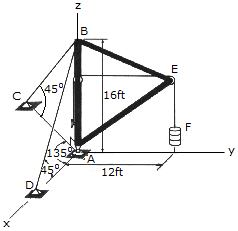# Engineering Mechanics - Equilibrium of a Rigid Body - Discussion

### Discussion :: Equilibrium of a Rigid Body - General Questions (Q.No.3)

3.Determine the tension in the supporting cables BC and BD and the components of reaction at the ball-and-socket joint A of the boom. The boom supports a drum having a weight of 200 lb. at F. Points C and D lie in the x—y plane.

 [A]. Ax = 0, Ay = 150 lb, Az = 562 lb, TBC = 300 lb, TBD = 212 lb [B]. Ax = 0, Ay = 150 lb, Az = 456 lb, TBC = 150 lb, TBD = 212 lb [C]. Ax = 0, Ay = 267 lb, Az = 843 lb, TBC = 533 lb, TBD = lb [D]. Ax = 0, Ay = 150 lb, Az = 500 lb, TBC = 212 lb, TBD = 212 lb

Explanation:

No answer description available for this question.

 Abshir said: (Jan 28, 2016) I need the solution.

 Nijanthan said: (Feb 21, 2016) I need working solution.

 Lasaca said: (Sep 25, 2017) Explain the solution please.